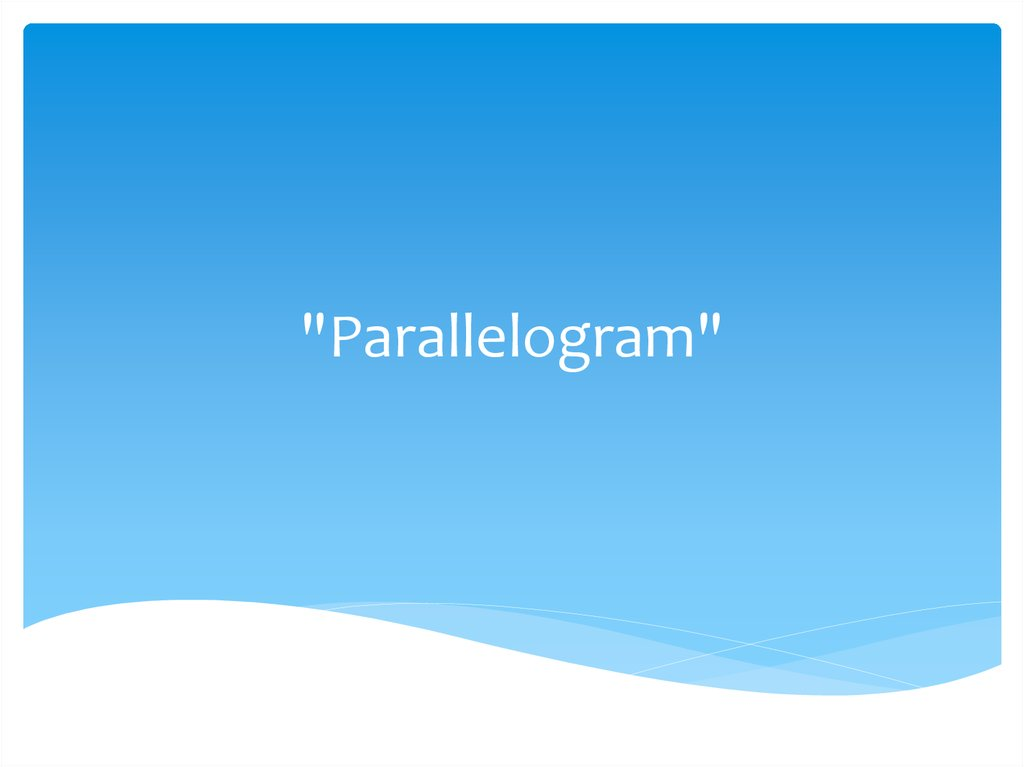# Parallelogram

"Parallelogram"

## 2. Lesson purpose

Educational:to study definition and a sign of a
parallelogram, to learn to build a parallelogram;
Developing:education of sense of beauty, interest in a
subject, collectivism, mutual aid.
Cultivate:development of logical thinking, creative
thinking, ability to analyze, development of spatial
representations, the mathematical speech.

## 3. Plan of the lesson:

1. Definition
2. Properties and sign

## 4. Definition of parallelogram

The parallelogram is a quadrangle at which the
opposite sides are in pairs parallel (lie on parallel
straight lines).

## 5. Properties of a parallelogram

• The opposite sides are equal;
• opposite corners, are also equal;;
• the sum of the corners adjacent to one party equals 180 degrees;
• the sum of all corners will be 360 degrees;
• diagonals are crossed, and divided by a point of intersection in half;
• diagonals divide a parallelogram into 2 triangles which are equal
among themselves;
• the point of intersection of diagonals will be his center of symmetry;
• the corner between heights will be equal to his acute angle;
• bisectors of 2 opposite corners are parallel.

## 6. Parallelogram signs

1. When the quadrangle has the parties from which
two equal and two parallel, this quadrangle will be a
parallelogram;
2. In case the quadrangle has in pairs equal opposite
sides, then it is a parallelogram;
3. Also, this figure will be a parallelogram when at a
quadrangle of his diagonal are crossed, and the point
of intersection divides them in half.

## 7. Parallelogram diagonals

Diagonal of a parallelogram is called any piece
connecting two tops of opposite corners of a
parallelogram.
The parallelogram has two diagonals - long d1, and
short - d2

## 8. Parallelogram perimeter

Perimeter of a parallelogram is called the sum of
lengths of all parties of a parallelogram

## 9. Area of a parallelogram

The area of a parallelogram is called the space limited
by the parties of a parallelogram, i.e. within
parallelogram perimeter.

## 11.

One of corners of a parallelogram is equal 65 °. To find other corners of a
parallelogram.
Decision.
∠C = ∠ A = 65 ° as opposite corners of a parallelogram.
∠А + ∠ In = 180 ° as corners, adjacent to one party of a parallelogram.
∠В = 180 ° — ∠А = 180 ° — 65 ° = 115 °.
∠D = ∠ B = 115 ° as opposite corners of a parallelogram.
Answer: ∠А = ∠ With = 65 °; ∠В = ∠ D = 115 °.